In this post we will learn how to apply our data science skills to solve a business problem – namely why passwords get stolen or hijacked?
This post is inspired from a blog entry on Data Science Central, where the solution was coded in Python. (Our analysis will use R programming and extend the original idea)

In this tutorial, we will explore the following questions:

1. What are the most common patterns found in passwords?
2. How many passwords are  banking type “strong ” combinations (containing special characters, length >8) ?
3. How many passwords make excessive use of repetitive characters, like “1111”, “007”, “aaabbbccc” or similar.

Remember, this is a “real-world” dataset and this type of list is often used to create password dictionaries. You can also use it to develop your own password strength checker.

Overall, this tutorial will cover the following topics:

1. basic string functions: stringlength, stringsearch, etc.
2. data visualization using pie charts, histograms,
3. Color coded HTML tables (similar to Excel) – a great feature if you plan to create Shiny Webapps with Tables.
4. Weighted ranking.

So let’s get started:

## What makes a “Strong” password?

First let us take a look at the minimum requirements of  an ideal password:

1. Minimum 8 characters in length.
2. Contains 3 out of 4 of the following items:
• Uppercase Letters
• Lowercase Letters
• Numbers
• Symbols

## Analysis Procedure:

1. Load input (password data) file:

TFscores = data.frame(fread(“C:/anu/ja/dec2016/passwords_data.txt”, stringsAsFactors = FALSE, sep = ‘\n’, skip = 16))

2. Calculate length of each password:

TFscores\$len = str_length(TFscores\$password)

3. Plot histogram to see frequency distribution of password lengths. Note, we use a custom for-loop to generate labels for the histogram.

hist(TFscores\$len, col = “blue” , ylim = c(0, 150000),

main = “Frequency Distribution – password length”,

xlab = “Password Length”,  ylab = “Count / Frequency”, labels = lendf\$labelstr)Histogram for password lengths

4.

a. Calculate number of digits in each password.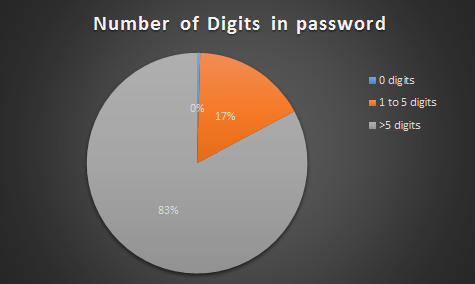number of digits in password

TFscores\$strmatch = gsub(pattern = “[[:digit:]]”, replacement = “”, TFscores\$password)

TFscores\$numberlen = TFscores\$len – str_length(TFscores\$strmatch)

b. Similarly calculate number of characters from other character classes:

• Upper case alphabets
• Lower case alphabets
• Special characters – ! â # % & â ( ) * + , – . / : ;

5. Assign 1 point as password strength “rank” for every character class present in the password.  As mentioned earlier, an ideal password should have at least 3 character classes.

TFscores\$rank = TFscores\$urank + TFscores\$lrank + TFscores\$nrank +   TFscores\$srank

Let us take a look to see how the passwords in our list stack up:

pie(piedfchar\$Var1,labels = labelarrchar , col=rainbow(9),  main=”no. of Character classes in password”)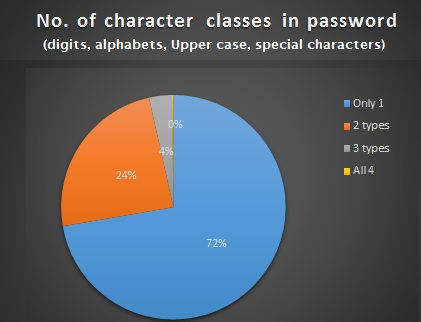password strength analysis

6. Count number of unique characters in password :

Note, this function is resource intensive, and takes couple of hours to complete due to size of the dataset.
To reduce the time/effort , the calculated values are added to the zipfolder, titled “pwd_scores.csv”.

length(unique(strsplit(tempx\$password, “”)[]))

7. Assign  password strength category based on rank and length:

TFscores\$pwdclass = “weak”   #default

TFscores\$pwdclass[TFscores\$len < 5 | TFscores\$rank == 1 ] = “very weak”

TFscores\$pwdclass[TFscores\$len >= 8 & TFscores\$rank >=2] = “medium”

TFscores\$pwdclass[TFscores\$len >= 12] = “strong”

TFscores\$pwdclass[TFscores\$len >= 12 & TFscores\$rank == 4] = “very strong”

Based on this criteria, we get the following frequency distribution: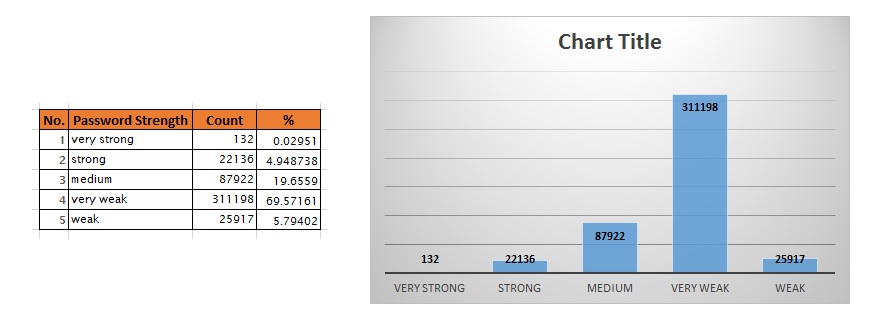password strength

7. We can derive the following insights from steps 5 and 6:

• 77.68% of passwords are weak or very weak!
• ~3% of passwords have less than 5 characters.
• ~72% of passwords have less only 1 type of character class.
• 0.5% of passwords have 8+ characters yet number of unique characters is less than 30%.
• ~0.9% of characters have less than 4 unique characters.
• 72% of passwords contain only digits.

8. Let’s see if there are any patterns repeated in the passwords, like “12345”, “abcde”, “1111”, etc:

TFscores\$strmatch = regexpr(“12345”, TFscores\$password)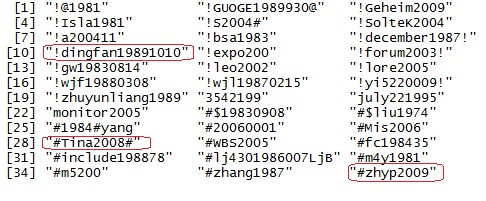password with year prefixes.

• 1.2% of passwords contain pattern “12345”.
• 0.01% of passwords contain pattern “abcde”.
• 0.3% of passwords contain pattern “1111”.
• 0.02% of passwords contain pattern “1234”.
• 15% of passwords contain year notations like “198*”, “197*”, “199”, “200*”. Sample shown alongside clearly shows that many people use important years from their life for their passwords. (logically true!)

9. View the password strength visually. We use the “condformat” function to create an HTML table that is easy to assimilate:

condformat(testsampledf) +  rule_fill_discrete(password, expression = rank < 2, colours = c(“TRUE”=”red”)) +
rule_fill_discrete(len, expression = (len >= 12), colours = c(“TRUE”=”gold”)) +
rule_fill_discrete(pwdclass, expression = (rank>2 & len>=8) , colours = c(“TRUE”=”green”))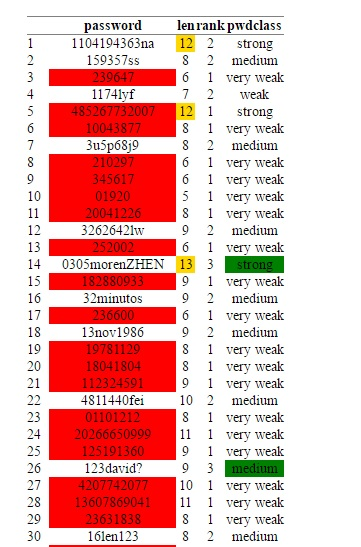password strength HTMl table